### Levers and Torque

#### Purposes

• Explore levers
• Calculate torque

#### Set Up

• A meter stick
• A spring scale
• String
• A mass.

#### Theory

Levers utilize torque to assist us in lifting or moving objects. Torque is the cross product between a force and the distance of the force from a fulcrum (the central point about which the system turns). The cross product takes only the component of the force acting perpendicular to the distance. Using trigonometry the torque is defined as:

Torque = Force × Distance to fulcrum × sin (θ)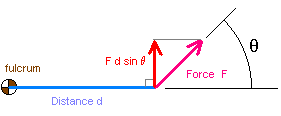Remember that work was also force multiplied by the distance, but it was the dot product and used the cosine of the angle between the force and distance: force × distance × cos(θ).

In this laboratory the force will be perpendicular (90°) to the distance. The sine of 90° is one, therefore the torque will be:

Torque = Force × Distance to fulcrum × sin (θ)
Torque = Force × Distance to fulcrum × sin (90°)
Torque = Force × Distance to fulcrum × 1
Torque = Force × Distance to fulcrum

#### Procedure, Data collection, and Calculations

##### Class I Levers trial one: de = dr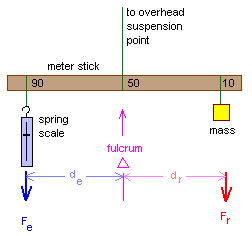In a class one lever the fulcrum is between the force of the resistance (Fr) and the force of the effort (Fe). In a class one lever the force of the effort (Fe) multiplied by the distance of the effort from the fulcrum (de) is equal to the force of the resistance (Fr) multiplied by the distance of the resistance from the fulcrum (dr). The effort and the resistance are on opposite sides of the fulcrum. Pliers are an example of a class one lever.

In the diagram, the mass provides the resistance, the spring scale measures our efforts. The spring scale is calibrated in grams. Grams are not a unit of force per se, but in this laboratory we will use the term gram-force" as the force exerted on one gram at the Earth's surface by the acceleration of gravity. One "gram-force" will be equivalent to 980 cm/sec2 (dynes).

For the diagram: Fe × de = Fr × dr

1. Hang a 200 gram mass at the 10 cm mark, hang the spring scale at the 90 cm mark, suspend the meter stick from the 50 cm mark.
2. Find Fe, de, Fr, dr in gram force. To determine the gram-force of the mass (Fr) use a balance beam scale. de and dr should be 40 cm if set up correctly. Fe can be read from the spring gram scale directly.
3. Calculate Fe × de and Fr × dr.
4. State whether Fe × de = Fr × dr.
5. Calculate the mechanical advantage Fr/Fe.
Fe de Fe × de Fr dr Fr × dr Fede = Frdr? M.A.
________ ________ ________ ________ ________ ________ Yes | No ________
##### Class I Levers trial two: de > dr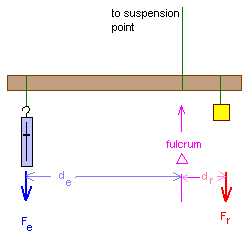For the diagram: Fe × de = Fr × dr

1. Switch masses to a 500 gram mass or two 200 gram masses lashed together.
2. Put the 500 gram mass at the 10 cm mark and the spring scale at the 90 cm mark, suspend the meter stick from the 30 cm mark.
3. Find Fe, de, Fr, dr in gram force.
4. Calculate Fe × de and Fr × dr.
5. State whether Fe × de = Fr × dr.
6. Calculate the mechanical advantage Fr/Fe.
Fe de Fe × de Fr dr Fr × dr Fede = Frdr? M.A.
________ ________ ________ ________ ________ ________ Yes | No ________
##### Class II Levers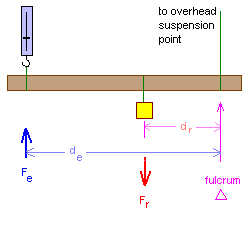In a class two lever the resistance is between the force of the effort and the fulcrum. In a class two lever the force of the effort multiplied by the distance of the effort from the fulcrum is opposite and equal to the force of the resistance multiplied by the distance of the resistance from the fulcrum. The effort and the resistance are on the same side of the fulcrum but point in opposite directions.

The effort distance (also sometimes called the "effort arm") is longer than the resistance distance.

Wheelbarrows and giant taro digging poles (when we push up on the pole) are examples of class two levers.

Note that our choice of down as being positive in the first part of the lab means that up is now negative in this section. So Fe is a negative force. Write Fe as negative in the table and then -Fe × de will be positive.

For the diagram: -Fe × de = Fr × dr
Mechanical advantage = |Fr/Fe | where | means "absolute value." Mechanical advantage is always positive.

1. Move the 500 gram mass (or two 200 gram masses) to around the 30 cm mark and the spring scale at the 90 cm mark, suspend the meter stick from the 10 cm mark. You may have to adjust the position of your mass according to the ability of your spring scale to provide an accurate reading. You want to avoid having to read either very small gram forces or gram forces too large for your spring scale. If you adjust positions, remember to measure the actual de and dr you are using!
2. Find Fe, de, Fr, dr in gram force.
3. Calculate Fe × de and Fr × dr.
4. State whether -Fe × de = Fr × dr.
5. Calculate the mechanical advantage Fr/Fe.
Fe de -Fe × de Fr dr Fr × dr -Fede = Frdr? M.A.
________ ________ ________ ________ ________ ________ Yes | No ________
##### Class III Levers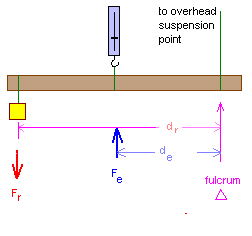In a class three lever the resistance is between the force of the effort and the fulcrum. In a class three lever the force of the effort multiplied by the distance of the effort from the fulcrum is opposite and equal to the force of the resistance multiplied by the distance of the resistance from the fulcrum. The effort and the resistance are on the same side of the fulcrum but point in opposite directions.

The effort distance (also sometimes called the "effort arm") is shorter than the resistance distance.

For the diagram: -Fe × de = Fr × dr
Mechanical advantage = |Fr/Fe | where | means "absolute value." Mechanical advantage is always positive.

1. Switch to a 100 gram mass.
2. Move the 100 gram mass to the 90 cm mark and the spring scale to somewhere around the 65 cm to 70 cm mark, keeping the meter stick suspended from the 10 cm mark. Once again adjust your spring scale and mass positions, if necessary, to get accurate readings from the spring scale.
3. Find Fe, de, Fr, dr in gram force.
4. Calculate Fe × de and Fr × dr.
5. State whether -Fe × de = Fr × dr.
6. Calculate the mechanical advantage Fr/Fe.
Fe de -Fe × de Fr dr Fr × dr -Fede = Frdr? M.A.
________ ________ ________ ________ ________ ________ Yes | No ________

In the class III lever the mechanical advantage might be called a mechanical disadvantage. Why? (Suggestion: Consider the force of the effort, is it less than the force of the resistance or more than the force of the resistance?)

Note that the human lower arm is a third class lever: the bicep, attached just below the elbow, can be used to lift a weight being held in the hand at the end of the lower arm.

##### Continuous Levers: Screwdrivers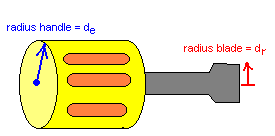A screwdriver is actually a form of lever where a handle with a large radius provides a mechanical advantage in turning a blade with a smaller radius. All sorts of circular devices make use of this form of mechanical advantage. Circular water valve handles, tire irons, socket wrenches, monkey wrenches, and many other items utilize this time of circular lever.

Measure the radius of the handle on a screwdriver and then measure the radius of the blade. Calculate the mechanical advantage from de/dr.

Note that the mechanical advantage to a circular device is de/dr while the mech. adv. for a lever was Fr/Fe. Note that the seeming "flip-flop" of the fraction is not a mistake.

Consider that Fe × de = Fr × dr. Cross dividing by Fe and dr yields:

```de = Fr